Add quantity values, doses, or variants to `SimBiology.Scenarios` object

Since R2019b

## Syntax

``sObj = add(sObj,combination,name,content)``
``sObj = add(sObj,combination,quantityNames,probDist,Name,Value)``
``sObj = add(sObj,combination,sObj2)``

## Description

example

````sObj = add(sObj,combination,name,content)` adds an entry to the `SimBiology.Scenarios` object `sObj`. The input argument `name` is the entry name and `content` is the entry content. `combination` specifies how to combine the new entry with the existing entries of `sObj`.```

example

````sObj = add(sObj,combination,quantityNames,probDist,Name,Value)` specifies to generate the sample values for one or more model quantities `quantityNames` from the joint probability distribution `probDist`. Specify additional options for the probability distributions and sampling method using one or more name-value pair arguments. To use probability distributions, you must have Statistics and Machine Learning Toolbox™.```

example

````sObj = add(sObj,combination,sObj2)` adds entries from the `SimBiology.Scenarios` object `sObj2` to `sObj`. The function combines the entries from `sObj2` with the existing entries from `sObj` using the specified `combination` method.```

## Examples

collapse all

Load the model of glucose-insulin response. For details about the model, see the Background section in Simulate the Glucose-Insulin Response.

`sbioloadproject('insulindemo','m1');`

The model contains different parameter values and initial conditions that represents different insulin impairments (such as Type 2 diabetes, low insulin sensitivity, and so on) stored in five variants.

`variants = getvariant(m1)`
```variants = SimBiology Variant Array Index: Name: Active: 1 Type 2 diabetic false 2 Low insulin se... false 3 High beta cell... false 4 Low beta cell ... false 5 High insulin s... false ```

Suppress an informational warning that is issued during simulations.

`warnSettings = warning('off','SimBiology:DimAnalysisNotDone_MatlabFcn_Dimensionless');`

Select a dose that represents a single meal of 78 grams of glucose.

`singleMeal = sbioselect(m1,'Name','Single Meal');`

Create a `Scenarios` object to represent different initial conditions combined with the dose. That is, create a `scenario` object where each variant is paired (or combined) with the dose, for a total of five simulation scenarios.

```sObj = SimBiology.Scenarios; add(sObj,'cartesian','variants',variants); add(sObj,'cartesian','dose',singleMeal)```
```ans = Scenarios (5 scenarios) Name Content Number ________ ___________________ ______ Entry 1 variants SimBiology variants 5 x Entry 2 dose SimBiology dose 1 See also Expression property. ```

`sObj` contains two entries. Use the `generate` function to combine the entries and generate five scenarios. The function returns a scenarios table, where each row represents a scenario and each column represents an entry of the `Scenarios` object.

`scenariosTbl = generate(sObj)`
```scenariosTbl=5×2 table variants dose ______________________ _________________________ 1x1 SimBiology.Variant 1x1 SimBiology.RepeatDose 1x1 SimBiology.Variant 1x1 SimBiology.RepeatDose 1x1 SimBiology.Variant 1x1 SimBiology.RepeatDose 1x1 SimBiology.Variant 1x1 SimBiology.RepeatDose 1x1 SimBiology.Variant 1x1 SimBiology.RepeatDose ```

Change the entry name of the first entry.

`rename(sObj,1,'Insulin Impairements')`
```ans = Scenarios (5 scenarios) Name Content Number ____________________ ___________________ ______ Entry 1 Insulin Impairements SimBiology variants 5 x Entry 2 dose SimBiology dose 1 See also Expression property. ```

Create a `SimFunction` object to simulate the generated scenarios. Use the `Scenarios` object as the input and specify the plasma glucose and insulin concentrations as reponses (outputs of the function to be plotted). Specify `[]` for the dose input argument since the `Scenarios` object already has the dosing information.

`f = createSimFunction(m1,sObj,{'[Plasma Glu Conc]','[Plasma Ins Conc]'},[])`
```f = SimFunction Parameters: Name Value Type Units _________________________ ______ _____________ ___________________________________________ {'Plasma Volume (Glu)' } 1.88 {'parameter'} {'deciliter' } {'k1' } 0.065 {'parameter'} {'1/minute' } {'k2' } 0.079 {'parameter'} {'1/minute' } {'Plasma Volume (Ins)' } 0.05 {'parameter'} {'liter' } {'m1' } 0.19 {'parameter'} {'1/minute' } {'m2' } 0.484 {'parameter'} {'1/minute' } {'m4' } 0.1936 {'parameter'} {'1/minute' } {'m5' } 0.0304 {'parameter'} {'minute/picomole' } {'m6' } 0.6469 {'parameter'} {'dimensionless' } {'Hepatic Extraction' } 0.6 {'parameter'} {'dimensionless' } {'kmax' } 0.0558 {'parameter'} {'1/minute' } {'kmin' } 0.008 {'parameter'} {'1/minute' } {'kabs' } 0.0568 {'parameter'} {'1/minute' } {'kgri' } 0 {'parameter'} {'1/minute' } {'f' } 0.9 {'parameter'} {'dimensionless' } {'a' } 0 {'parameter'} {'1/milligram' } {'b' } 0.82 {'parameter'} {'dimensionless' } {'c' } 0 {'parameter'} {'1/milligram' } {'d' } 0.01 {'parameter'} {'dimensionless' } {'kp1' } 2.7 {'parameter'} {'milligram/minute' } {'kp2' } 0.0021 {'parameter'} {'1/minute' } {'kp3' } 0.009 {'parameter'} {'(milligram/minute)/(picomole/liter)' } {'kp4' } 0.0618 {'parameter'} {'(milligram/minute)/picomole' } {'ki' } 0.0079 {'parameter'} {'1/minute' } {'[Ins Ind Glu Util]' } 1 {'parameter'} {'milligram/minute' } {'Vm0' } 2.5129 {'parameter'} {'milligram/minute' } {'Vmx' } 0.047 {'parameter'} {'(milligram/minute)/(picomole/liter)' } {'Km' } 225.59 {'parameter'} {'milligram' } {'p2U' } 0.0331 {'parameter'} {'1/minute' } {'K' } 2.28 {'parameter'} {'picomole/(milligram/deciliter)' } {'alpha' } 0.05 {'parameter'} {'1/minute' } {'beta' } 0.11 {'parameter'} {'(picomole/minute)/(milligram/deciliter)'} {'gamma' } 0.5 {'parameter'} {'1/minute' } {'ke1' } 0.0005 {'parameter'} {'1/minute' } {'ke2' } 339 {'parameter'} {'milligram' } {'Basal Plasma Glu Conc'} 91.76 {'parameter'} {'milligram/deciliter' } {'Basal Plasma Ins Conc'} 25.49 {'parameter'} {'picomole/liter' } Observables: Name Type Units _____________________ ___________ _______________________ {'[Plasma Glu Conc]'} {'species'} {'milligram/deciliter'} {'[Plasma Ins Conc]'} {'species'} {'picomole/liter' } Dosed: TargetName TargetDimension __________ _____________________ {'Dose'} {'Mass (e.g., gram)'} TimeUnits: hour ```

Simulate the model for 24 hours and plot the simulation data. The data contains five runs, where each run represents a scenario in the Scenarios object.

```sd = f(sObj,24); sbioplot(sd)```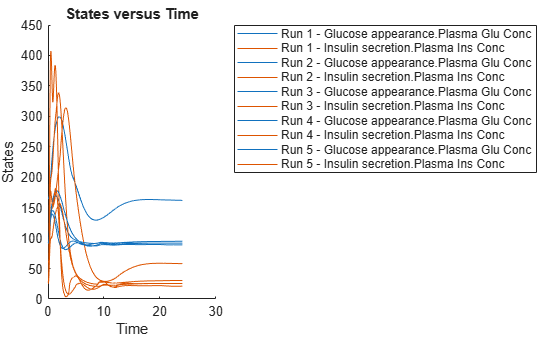```ans = Axes (SbioPlot) with properties: XLim: [0 30] YLim: [0 450] XScale: 'linear' YScale: 'linear' GridLineStyle: '-' Position: [0.0956 0.1100 0.2509 0.8150] Units: 'normalized' Show all properties ```

If you have Statistics and Machine Learning Toolbox™, you can also draw sample values for model quantities from various probability distributions. For instance, suppose that the parameters `Vmx` and `kp3`, which are known for the low and high insulin sensitivity, follow the lognormal distribution. You can generate sample values for these parameters from such a distribution, and perform a scan to explore model behavior.

Define the lognormal probability distribution object for `Vmx`.

`pd_Vmx = makedist('lognormal')`
```pd_Vmx = LognormalDistribution Lognormal distribution mu = 0 sigma = 1 ```

By definition, the parameter `mu` is the mean of logarithmic values. To vary the parameter value around the base (model) value of the parameter, set `mu` to `log(``model_value``)`. Set the standard deviation (sigma) to 0.2. For a small sigma value, the mean of a lognormal distribtion is approximately equal to `log(``model_value``)`. For details, see Lognormal Distribution (Statistics and Machine Learning Toolbox).

```Vmx = sbioselect(m1,'Name','Vmx'); pd_Vmx.mu = log(Vmx.Value); pd_Vmx.sigma = 0.2```
```pd_Vmx = LognormalDistribution Lognormal distribution mu = -3.05761 sigma = 0.2 ```

Similarly define the probability distribution for kp3.

```pd_kp3 = makedist('lognormal'); kp3 = sbioselect(m1,'Name','kp3'); pd_kp3.mu = log(kp3.Value); pd_kp3.sigma = 0.2```
```pd_kp3 = LognormalDistribution Lognormal distribution mu = -4.71053 sigma = 0.2 ```

Now define a joint probability distribution to draw sample values for Vmx and kp3, with a rank correlation to specify some correlation between these two parameters. Note that this correlation assumption is for the illustration purposes of this example only and may not be biologically relevant.

First remove the variants entry (entry 1) from `sObj`.

`remove(sObj,1)`
```ans = Scenarios (1 scenarios) Name Content Number ____ _______________ ______ Entry 1 dose SimBiology dose 1 See also Expression property. ```

Add an entry that defines the joint probability distribution with a rank correlation matrix.

`add(sObj,'cartesian',["Vmx","kp3"],[pd_Vmx, pd_kp3],'RankCorrelation',[1,0.5;0.5,1])`
```ans = Scenarios (2 scenarios) Name Content Number ____ ______________________ ___________ Entry 1 dose SimBiology dose 1 x (Entry 2.1 Vmx Lognormal distribution 2 (default) + Entry 2.2) kp3 Lognormal distribution 2 (default) See also Expression property. ```

By default, the number of samples to draw from the joint distribution is set to 2. Increase the number of samples.

`updateEntry(sObj,2,'Number',50)`
```ans = Scenarios (50 scenarios) Name Content Number ____ ______________________ ______ Entry 1 dose SimBiology dose 1 x (Entry 2.1 Vmx Lognormal distribution 50 + Entry 2.2) kp3 Lognormal distribution 50 See also Expression property. ```

Verify that the `Scenarios` object can be simulated with the model. The `verify` function throws an error if any entry does not resolve uniquely to an object in the model or the entry contents have inconsistent lengths (sample sizes). The function throws a warning if multiple entries resolve to the same object in the model.

`verify(sObj,m1)`

Generate the simulation scenarios. Plot the sample values using `plotmatrix`. You can see the value of `Vmx` is varied around its model value 0.047 and that of `kp3` around 0.009.

```sTbl = generate(sObj); [s,ax,bigax,h,hax] = plotmatrix([sTbl.Vmx,sTbl.kp3]); ax(1,1).YLabel.String = "Vmx"; ax(2,1).YLabel.String = "kp3"; ax(2,1).XLabel.String = "Vmx"; ax(2,2).XLabel.String = "kp3";```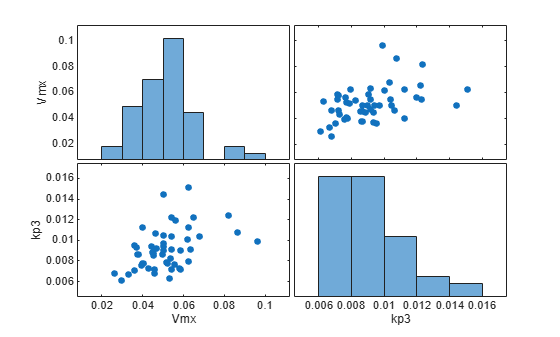Simulate the scenarios using the same SimFunction you created previously. You do not need to create a new SimFunction object even though the Scenarios object has been updated.

```sd2 = f(sObj,24); sbioplot(sd2);```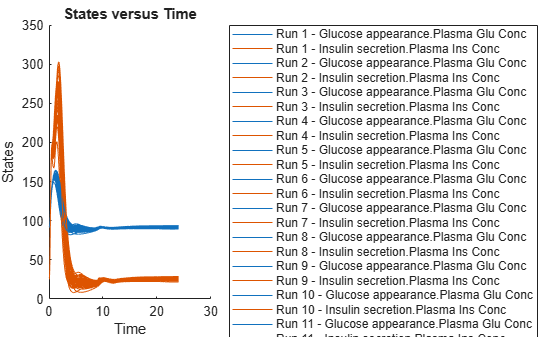By default, SimBiology uses the random sampling method. You can change it to the Latin hypercube sampling (or sobol or halton) for a more systematic space-filling approach.

`entry2struct = getEntry(sObj,2)`
```entry2struct = struct with fields: Name: {'Vmx' 'kp3'} Content: [2x1 prob.LognormalDistribution] Number: 50 RankCorrelation: [2x2 double] Covariance: [] SamplingMethod: 'random' SamplingOptions: [0x0 struct] ```
`entry2struct.SamplingMethod = 'lhs'`
```entry2struct = struct with fields: Name: {'Vmx' 'kp3'} Content: [2x1 prob.LognormalDistribution] Number: 50 RankCorrelation: [2x2 double] Covariance: [] SamplingMethod: 'lhs' SamplingOptions: [0x0 struct] ```

You can now use the updated structure to modify entry 2.

`updateEntry(sObj,2,entry2struct)`
```ans = Scenarios (50 scenarios) Name Content Number ____ ______________________ ______ Entry 1 dose SimBiology dose 1 x (Entry 2.1 Vmx Lognormal distribution 50 + Entry 2.2) kp3 Lognormal distribution 50 See also Expression property. ```

Visualize the sample values.

```sTbl2 = generate(sObj); [s,ax,bigax,h,hax] = plotmatrix([sTbl2.Vmx,sTbl2.kp3]); ax(1,1).YLabel.String = "Vmx"; ax(2,1).YLabel.String = "kp3"; ax(2,1).XLabel.String = "Vmx"; ax(2,2).XLabel.String = "kp3";```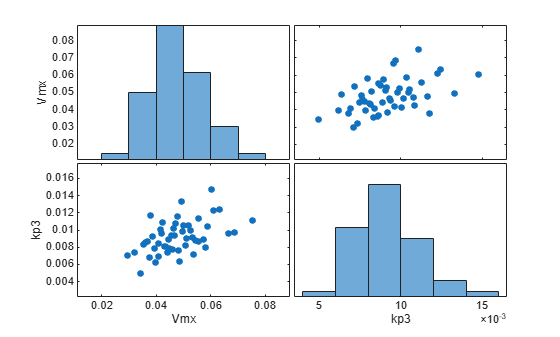Simulate the scenarios.

```sd3 = f(sObj,24); sbioplot(sd3);```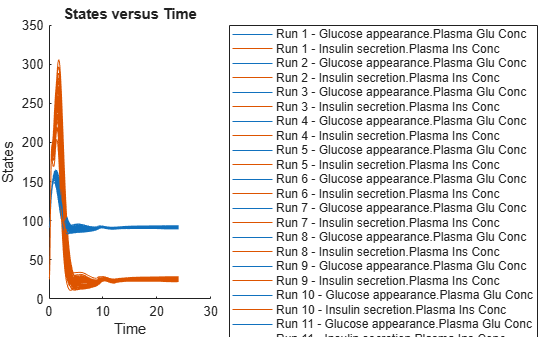Restore warning settings.

`warning(warnSettings);`

## Input Arguments

collapse all

Simulation scenarios, specified as a `SimBiology.Scenarios` object.

Simulation scenarios, specified as a `SimBiology.Scenarios` object.

Entry name, specified as a character vector or string.

You can set the entry name to the name of a model quantity (species, parameter, or compartment). Alternatively, you can define a name for a group of doses or variants to be included in the sample (scenarios) generation.

Example: `"k1"`

Data Types: `char` | `string`

Model quantity values, or a vector of doses or variants, specified as a numeric vector, vector of `RepeatDose` or `ScheduleDose` objects, or vector of `variant` objects.

If you specify a quantity name for the `name` input argument, set `content` to a numeric vector.

If you specify a name for a group of doses or variants, set `content` to a vector of dose objects or vector of variant objects.

Example: `[0.5,1,1.5]`

Method to combine entries, specified as one of the following:

• `'cartesian'` – Combine entries by taking the Cartesian product of the corresponding sample values. This is denoted by the cross symbol `x`.

• `'elementwise'` – Combine entries one to one (elementwise), that is, the first element from the first entry is paired with the first element from the second entry and so on. This is denoted by the plus symbol `+`. The entries must have the same number of sample values (elements) for this method.

For details, see Combine Simulation Scenarios in SimBiology.

Names of model quantities for the sample (scenario) generation, specified as a character vector, string, string vector, or cell array of character vectors.

Example: `["k12","k21"]`

Data Types: `char` | `string` | `cell`

Probability distributions to generate sample values for model quantities, specified as a vector of probability distribution objects, character vector, string, string vector, or cell array of character vectors containing the names of supported probability distributions. To specify the probability distributions, you must have Statistics and Machine Learning Toolbox.

Use the `makedist` (Statistics and Machine Learning Toolbox) function to create distribution objects. For a list of supported distributions, see distname (Statistics and Machine Learning Toolbox).

Example: `[pd1,pd2]`

### Name-Value Arguments

Specify optional pairs of arguments as `Name1=Value1,...,NameN=ValueN`, where `Name` is the argument name and `Value` is the corresponding value. Name-value arguments must appear after other arguments, but the order of the pairs does not matter.

Before R2021a, use commas to separate each name and value, and enclose `Name` in quotes.

Example: `'Number',10` specifies to generate 10 samples.

Number of samples to draw from probability distributions, specified as the comma-separated pair consisting of `'Number'` and a positive scalar. The default value `[]` means that the function infers the number of samples from other entries. If the number cannot be inferred, the number is set to `2`.

Example: `'Number',5`

Rank correlation matrix for the joint probability distribution, specified as the comma-separated pair consisting of `'RankCorrelation'` and a numeric matrix. The default behavior is that when both `'RankCorrelation'` and `'Covariance'` are set to `[]`, `SimBiology.Scenarios` draws uncorrelated samples from the joint probability distribution.

You cannot specify `'RankCorrelation'` if `'Covariance'` is set. The number of columns in the matrix must match the number of specified distributions. The matrix must be symmetric with diagonal values of 1. All of its eigenvalues must also be positive.

Example: `'RankCorrelation',[1 0.3;0.3 1]`

Mean values of quantities, specified as the comma-separated pair consisting of `'Mean'` and a numeric vector.

You can specify mean values for normal distributions only. The number of mean values must equal the number of specified probability distributions.

Example: `'Mean',[0.5,1.5]`

Covariance matrix for the joint probability distribution, specified as the comma-separated pair consisting of `'Covariance'` and a numeric matrix. The default behavior is that if both `'RankCorrelation'` and `'Covariance'` are set to `[]`, `SimBiology.Scenarios` draws uncorrelated samples from the joint probability distribution. You cannot specify `'Covariance'` if you specify `'RankCorrelation'`.

You can specify the covariance matrix for normal distributions only. The number of columns in the matrix must match the number of specified distributions. All of its eigenvalues must also be nonnegative.

Example: `'Covariance',[0.25 0.15;0.15 0.25]`

Sampling method, specified as the comma-separated pair consisting of `'SamplingMethod'` and a character vector or string. Depending on whether probability distributions with `'RankCorrelation'` or normal distributions with `'Covariance'` are specified, the sampling techniques differ.

If an entry contains a (joint) normal distribution with `Covariance` specified, the sampling methods are:

• `'random'` – Draw random samples from the specified normal distribution using `mvnrnd` (Statistics and Machine Learning Toolbox).

• `'lhs'` – Draw Latin hypercube samples from the specified normal distributions using `lhsnorm` (Statistics and Machine Learning Toolbox). For details, see Generating Quasi-Random Numbers (Statistics and Machine Learning Toolbox).

If an entry contains a (joint) distribution with no `Covariance` specified, the sampling methods are:

• `'random'` – Draw random samples from the specified probability distributions using `random` (Statistics and Machine Learning Toolbox).

• `'lhs'` – Draw Latin hypercube samples from the specified probability distributions using an algorithm similar to `lhsdesign` (Statistics and Machine Learning Toolbox). This approach is a more systematic space-filling approach than random sampling. For details, see Generating Quasi-Random Numbers (Statistics and Machine Learning Toolbox).

• `'copula'` – Draw random samples using a copula (Statistics and Machine Learning Toolbox). Use this option to impose correlations between samples using copulas.

• `'sobol'` – Use the sobol sequence (`sobolset` (Statistics and Machine Learning Toolbox)) which is transformed using the inverse cumulative distribution function (`icdf` (Statistics and Machine Learning Toolbox)) of the specified probability distributions. Use this method for highly systematic space-filling. For details, see Generating Quasi-Random Numbers (Statistics and Machine Learning Toolbox).

• `'halton'` – Use the halton sequence (`haltonset` (Statistics and Machine Learning Toolbox)) which is transformed using the inverse cumulative distribution function (`icdf` (Statistics and Machine Learning Toolbox)) of the specified probability distributions. For details, see Generating Quasi-Random Numbers (Statistics and Machine Learning Toolbox).

If no `Covariance` is specified, `SimBiology.Scenarios` essentially performs two steps. The first step is to generate samples using one of the above sampling methods. For `lhs`, `sobol`, and `halton` methods, the generated uniform samples are transformed to samples from the specified distribution using the inverse cumulative distribution function `icdf` (Statistics and Machine Learning Toolbox). Then, as the second step, the samples are correlated using the Iman-Conover algorithm if `RankCorrelation` is specified. For `random`, the samples are drawn directly from the specified distributions and the samples are then correlated using the Iman-Conover algorithm.

Example: `'SamplingMethod','lhs'`

Options for the sampling method, specified as a scalar struct. The options differ depending on the sampling method: `sobol`, `halton`, or `lhs`.

For `sobol` and `halton`, specify each field name and value of the structure according to each name-value argument of the `sobolset` (Statistics and Machine Learning Toolbox) or `haltonset` (Statistics and Machine Learning Toolbox) function. SimBiology uses the default value of `1` for the `Skip` argument for both methods. For all other name-value arguments, the software uses the same default values of `sobolset` or `haltonset`. For instance, set up a structure for the `Leap` and `Skip` options with nondefault values as follows.

```s1.Leap = 50; s1.Skip = 0;```

For `lhs`, there are three samplers that support different sampling options.

• If you specify a covariance matrix, SimBiology uses `lhsnorm` (Statistics and Machine Learning Toolbox) for sampling. `SamplingOptions` argument is not allowed.

• Otherwise, use the field name `UseLhsdesign` to select a sampler.

• If the value is `true`, SimBiology uses `lhsdesign` (Statistics and Machine Learning Toolbox). You can use the name-value arguments of `lhsdesign` to specify the field names and values.

• If the value is `false` (default), SimBiology uses a nonconfigurable Latin hypercube sampler that is different from `lhsdesign`. This sampler does not require Statistics and Machine Learning Toolbox. `SamplingOptions` cannot contain any other options, except `UseLhsdesign`.

For instance, set up a structure to use `lhsdesign` with the `Criterion` and `Iterations` options.

```s2.UseLhsdesign = true; s2.Criterion = "correlation"; s2.Iterations = 10;```

Example: `'SamplingOptions',struct("Skip",5)`

Data Types: `struct`

## Output Arguments

collapse all

Simulation scenarios, returned as a `Scenarios` object.

## Version History

Introduced in R2019b